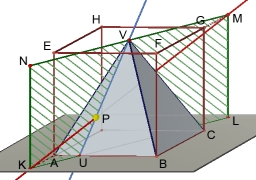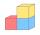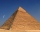# Height of pyramid

The pyramid ABCDV has edge lengths: AB = 4, AV = 7. What is its height?

h =  6.4031

### Step-by-step explanation:Did you find an error or inaccuracy? Feel free to write us. Thank you!Tips to related online calculators
Pythagorean theorem is the base for the right triangle calculator.

#### You need to know the following knowledge to solve this word math problem:

We encourage you to watch this tutorial video on this math problem:

## Related math problems and questions:

• Distance of pointsA regular quadrilateral pyramid ABCDV is given, in which edge AB = a = 4 cm and height v = 8 cm. Let S be the center of the CV. Find the distance of points A and S.
• Tetrahedral pyramidIt is given a regular tetrahedral pyramid with a base edge of 6 cm and the height of the pyramid 10 cm. Calculate the length of its side edges.
• CalculateCalculate the length of the wall diagonal of the cube with an edge 5 cm long.
• Hexagonal pyramidPlease calculate the height of a regular hexagonal pyramid with a base edge of 5cm and a wall height of w = 20cm. Please sketch a picture.
• The pyramidThe pyramid with a square base is 50 m high and the height of the sidewall is 80 m. Find the endge of the base of the pyramid.
• Regular 4-sided pyramidFind the area (surface area) of a regular 4-sided pyramid if its height is 20 m and the wall height is 23 m.
• The pyramid 4sThe pyramid with a rectangular base measuring 6 dm and 8 dm has a side edge of length 13 dm. Calculate the surface area and volume of this pyramid.
• Tetrahedral pyramidWhat is the surface of a regular tetrahedral (four-sided) pyramid if the base edge a=16 and height v=16?
• Body diagonalCuboid with base 7cm x 3,9cm and body diagonal 9cm long. Find the height of the cuboid and the length of the diagonal of the base,
• How toHow to find a total surface of a rectangular pyramid if each face is to be 8 dm high and the base is 10 dm by 6 dm.The regular quadrilateral pyramid has a base edge a = 1.56 dm and a height h = 2.05 dm. Calculate: a) the deviation angle of the sidewall plane from the base plane b) deviation angle of the side edge from the plane of the baseRegular tetrahedral pyramid has a base edge a=17 and collaterally edge length b=32. What is its height?2/4 of the area of ​​the roof-shaped regular tetrahedral pyramid with base edge 10 m and height of 4 m is already covered with roofing. How many square meters still need to be covered?The height of a regular pentagonal pyramid is as long as the edge of the base, 20 cm. Calculate the volume and surface area of the pyramid.Calculate the length of the square diagonal if the perimeter is 172 cm.Determine the length of the cube diagonal with edge 37 mm.Calculate the volume of a regular 6-sided cut pyramid if the bottom edge is 30 cm, the top edge is 12 cm, and the side edge length is 41 cm.